# HyperSpace, User Manual

Written by Paul Bourke
December 1990This document is the user manual for a program on the Macintosh for exploring 4 dimensional geometry. Here we will introduce the concepts of 4D geometry, describe the use of the application, and present some examples.

Introduction

 One dimensional space consists of the points along a line, only one number is required to uniquely describe any position in a 1D world.Two dimensional space consists of all the points on a plane, two numbers are required to uniquely describe any position in a 2D world. The two numbers used to describe any point can be considered to be positions on two noncolinear one dimensional axes, the particular axis system from all the possible combinations is usually chosen to be the one where the two axes are perpendicular to each other.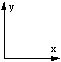For three dimensional space we add another axis perpendicular to the two used for the two dimensional space. This is what we use to represent the universe we live in, three numbers uniquely describe any point in our 3D universe and they are usually given the symbols x,y, and z.In four dimensions we "simply" need to add another coordinate axis that is perpendicular to the three axes used for 3D space. Most people have difficulty visualizing this but there is no problem mathematically and we can approach higher dimensions in the same way (although we won't do so here). We will use the symbol w to represent the coordinate axis of this 4th dimension. Any point then in four dimensional space can be represented by the four numbers w,x,y, and z.Hyperdimensional objects

The geometry of familiar objects in higher dimensions is well established mathematically but is generally outside our everyday experience. A mathematical approach will not be attempted here but rather the intuitive description of 4D geometric objects.

Generation by intuition

The creation of 4D geometric objects by intuition relies on being able to determine an progressive description of the equivalent object in 3D and how it was created from its 2D equivalent. If we "know" how the 3D object is created from 2D and maybe how the 2D object was created from 1D then the rules can be applied to the 3D object to form the 4D equivalent.
In what follows the rules for progressing each geometric primitive from 1 to 2 and then to 3 dimensions is explained. All the geometries below are implemented in the HyperSpace program, they can be explored there.

Cube

A one dimensional cube is a line segment. To create a 2D cube, a square. we "extrude" the line (1D cube) along the newly introduced axis. To form the 3D cube we again extrude the square (2D cube) along the newly introduced axis. So the general technique for generating a n+1 dimensional cube is take the n dimensional cube and extrude it along the new n+1 dimension.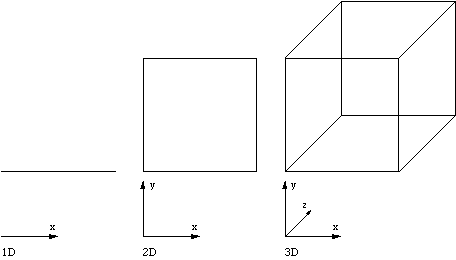Tetrahedron

1D tetrahedron is a line. 2D tetrahedron is an equilateral triangle. The n+1 dimension tetrahedron is created by taking the midpoint of the n dimensional tetrahedron and pulling it into the n+1 dimension.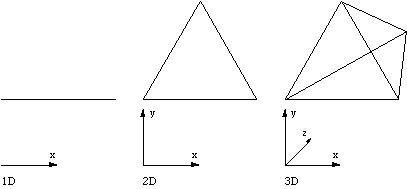Octahedron

1D octahedron is a line. 2D octahedron is a square. To form a n+1 dimensional octahedron take the midpoint of the n dimensional octahedron and pull it along the positive and negative axis of the n+1 dimension.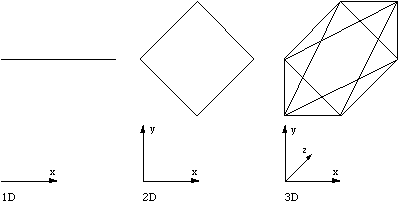Pyramid

To create a n+1 dimensional square based pyramid take the n dimensional cube and pull the midpoint into the n+1 dimension.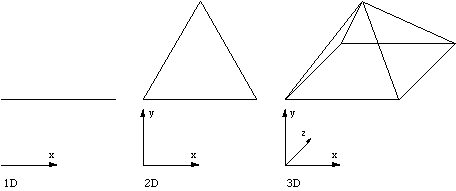Prism

A n+1 dimensional prism is the n dimensional cube with two opposite midpoints pulled into the n+1 dimension.Display techniques

The main visualization problem associated with 3D modelling is how to represent a 3D scene on a medium with one fewer dimensions, ie: a computer screen or piece of paper. This problem is made much more difficult for HyperSpace since now we need to represent 4D objects on a medium with two fewer dimensions. HyperSpace employs four fundamental techniques to solve this problem, they are discussed below.

Parallel Projections

In order to visualize 3D objects on a 2D medium it is common to simply ignore one coordinate. For example: ignore the z coordinate and draw the x and y coordinates. Such a technique gives what are called parallel projections and isometric projections of 3D objects.

This technique can be used to represent 4D objects on a 2D medium, however in this case we have to ignore two coordinates. For example: ignore the w and y coordinates and draw only the x and z coordinates. This is what the xy projection menu item in the view menu does. One should be very wary about trying to visualize the geometry of a 4D object by this technique. It is analogous to trying to visualize the geometry of a 3D cube by projections of it onto a line.

HyperCube examples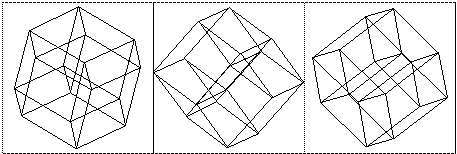Elevations and plans

Another technique employed to represent 3D objects on a 2D medium is to project the object onto each coordinate plane, xy, xz, and yz.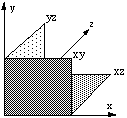This gives the common top/bottom (plan), front/back (elevation) and left/right views of a 3D object. For example a simple 3D structure is shown below with the projections onto the three coordinate planes.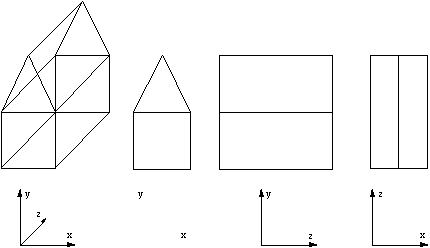This technique can be used for 4D objects except that there are six 2D projection planes, namely wx, wy, wz, xy, xz, and yz. Each of these can be displayed in the six views window shown below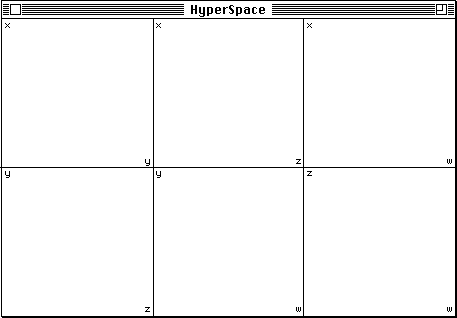Note that the axes labels are placed in the top-left and bottom-right of each view portion. The three view portions in the top left corner correspond to the top, front, and side views in 3D. For an unrotated HyperCube the six axis views are quite boring, they are all squares. The following shows the 6 axis views if the HyperCube is rotated on all planes.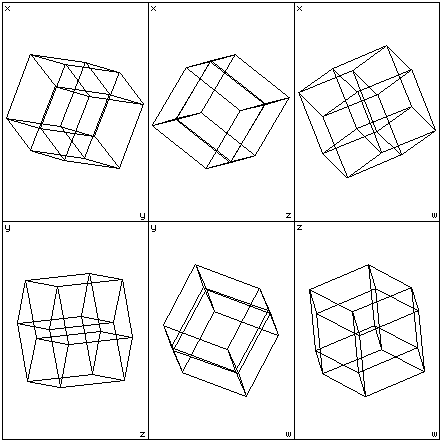Slices

The third technique used to represent 3D objects on a 2D medium is to contour the object, effectively cutting slices along one coordinate axis. It is usual in 3D to take the contours along the z axis but any axis will do. An arbitrary slicing plane may also be used or alternatively the contours can always be with respect to one axis and the object rotated in order to obtain arbitrary slicing planes. This process essentially removes one dimension, so slicing a 3D object results in a series of 2D curves. the contour lines. To contour 4D objects we will choose to always slice along the w axis. Removing one dimension from 4D objects results in a series of 3D objects which can be projected by any of the classical techniques.

The window for 9 slices is shown below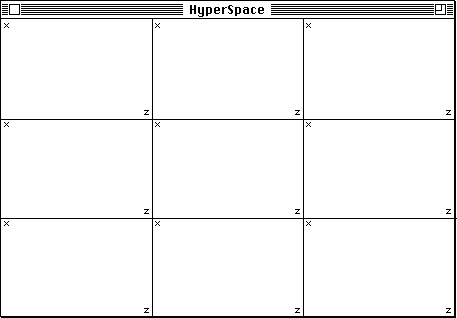The window for different numbers of slices simply partitions the window into smaller view portions. The axes labels are shown, the w axes is the one along which the slices are taken, the y axes is ignored (projected onto the x-z plane). Nine slices of the HyperCube are shown below. It doesn't seem that difficult to imagine each of the solids below.....the problem comes when you need to stack them up together in the 4th dimension.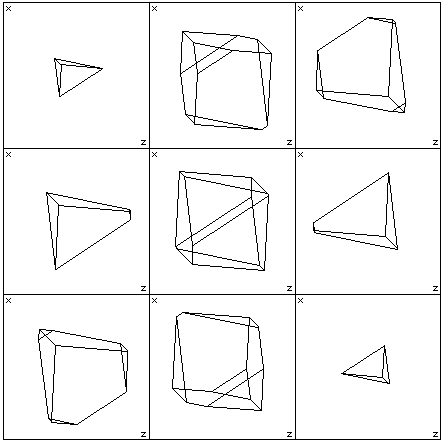Depth Cue

The last technique which can be used in conjunction the others is to shade the edges according to the value of one coordinate. In the HyperSpace application, points with a large y coordinate may be shaded red, points with a small y may be shaded blue and all points in between are coloured with the appropriate intermediate hue.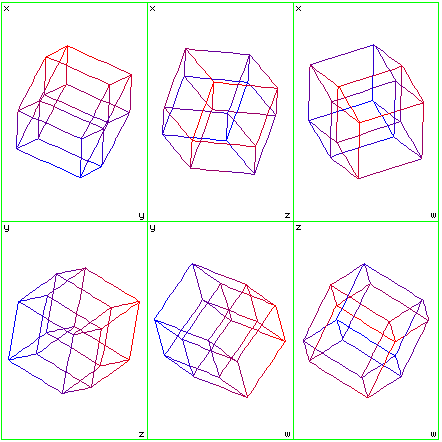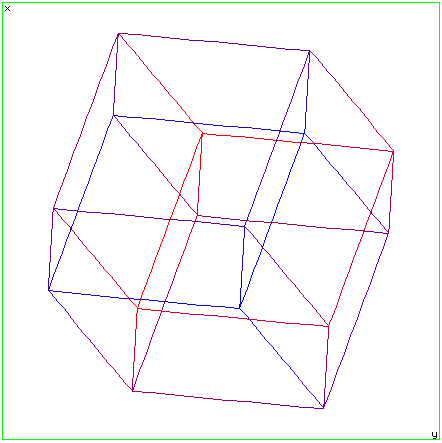Rotating the object

When viewing an object using a computer aided design package there are two operations which can be useful. They are: rotate the camera about the object or rotate the object about its centre. There are subtle differences between the two approaches, the HyperSpace application implements rotation of object about any one of the coordinate axis.

Considering the situation in 3D, there are three axes, x,y, and z about which an object can be rotated. Alternatively there can be considered to be three planes through which an object may be rotated. The two descriptions are identical, the only difference is in how they are described. So, for example, rotation about the x axis is the same as rotation in the y-z plane, rotation about the y axis is rotation in the x-z plane and rotation about the z axis is rotation in the x-y plane. The rotation plane is the terminology used here.

 In 4D there are six planes though which an object can be rotated. The amount of rotation in each plane is specified with the rotate controls window shown below. Each of the six sliders ranges from -180 degrees at the top to 180 degrees at the bottom. By clicking on the arrows the angle varies by one degree intervals, by clicking in the grey region the angle varies in 10 degree steps. If the slider reaches the bottom of the scale it will wrap around to the top and similarly when the slider reaches the top it will wrap around to the bottom, ie: -190 degree rotation = 180 degree rotation. With dimension set to 3 the first three sliders are disabled.It is possible to use HyperSpace to view other 4 dimensional objects. To do this a specially formatted text file must be created describing the geometry. This file is opened with the read menu item in the file menu. The format will be described below but it should be stressed that the format must be closely adhered to if HyperSpace is to correctly read the object geometry.

The text file consists of three sections, they are: the vertex description, the edge description, and finally the face description. Each section starts with the number of elements in that section, for example, the vertex section starts with the number of vertices that will follow, the edge section starts with the number of edges to follow, similarly for the faces.

Vertices: Each vertex appears on a line by itself and consists of four floating point numbers representing the w,x,y,z coordinates.

Edges: Each line of the edge description contains two numbers representing the vertex numbers of the endpoints of the edge.

Faces: Each line of the face description consists of five numbers. The first is the number of vertices in the face, it may be either 3 or 4. The remaining numbers are the vertex numbers making up the face. All four vertex numbers must be specified even if they are not used, the unused vertex number could be 0, it will be ignored in any case.

The vertices and edges must be present for any object description but the faces information is optional. If the faces are not specified then the number of faces must be set to 0. Note however that without faces the slicing method of visualization will not work.

This format is the one generated when the save menu item is used in the file menu. An example of a correctly formatted file is shown below

```5
-1 -1 -1 1
-1 1 1 1
-1 1 -1 -1
-1 -1 1 -1
1 0 0 0
10
0 1
0 2
0 3
1 2
1 3
2 3
4 0
4 1
4 2
4 3
10
3 0 1 2 0
3 0 1 3 0
3 0 2 3 0
3 1 2 3 0
3 4 0 1 0
3 4 1 2 0
3 4 1 3 0
3 4 0 2 0
3 4 0 3 0```

This object contains 5 vertices, 10 edges, and 10 faces. Note from the edge list that the vertex numbers start from 0 not 1, so the vertex numbers in the edge and face list range from 0 to 4 not from 1 to 5. The face list consists of only 3 point faces, the fourth column must be present but it is only considered for 4 point faces.

While all the numbers above are integers floating point numbers are equally valid. The separators between each number can be any "white" character, most commonly spaces or tabs would be used. This makes it possible to create these files with basic programs, spreadsheets, word processors, etc.

References

Experiments in 4 Dimensions, Heiserman, David L., TAB Books Inc

Regular Polytypes, Coxeter, H.S.M., MacMillam Company

Introduction to Geometry, Coxeter, H.S.M., John Wiley & Sons Inc

4 Dimensional Space, Eckhart, L., Indiana University Press

# Regular Polytopes (Platonic solids) in 4D

Written by Paul Bourke
June 1997
Update November 2003

 Quote from Ephesians 3, 17-18 (King James version) That ye ... may be able to comprehend with all the saints what is the breadth, and length, and depth, and height. Is this the first reference (New Testament of the Bible) to 4 dimensions?

Regular polytopes or platonic solids are convex solids (closed) where all the building blocks (vertices, edges, faces, hyperfaces) have the same characteristics. That is, vertices have the same number of neighbours, edges are all the same length, polygons are all the same shape and area, and hyperfaces have the same volume.

In 2 dimensions there an infinity of regular polytopes. For example, a circle can be approximated by polygons with 2 edges (line), 3 edges (triangle), 4 edges (square), 5 edges, 6 edges ..... indeed any number of edges. In 2 dimensions, every regular polytope is its own dual.

In 3 dimensions there are just 5, these are typically known as the platonic solids. In 3 dimensions, the tetrahedron is self-dual. The dual of the cube is the octahedron. And the dual of the dodecahedron is the icosahedron.

Regular polytopes in 4 Dimensions

In 4 dimensions there are 6 regular polytopes, this is the highest number that exist in any dimension greater than 2. They are listed and described in order of increasing cell numbers below. Each regular polytope is supplied as data in at least two versions, the first is a simple ASCII format listing vertices, edges, and faces. The second is as an OFF file ready for GeomView.

### Simplex

• Also known as pentatope or pentachoron
• Self-dual
• 4D equivalent of the tetrahedron
• 5 tetrahedral cells, 10 triangular faces, 10 edges, 5 vertices
• 3 tetrahedra meet at an edge
• It can be intuitively formed by choosing a point in one higher dimension equidistant to all the vertices in the current dimension and connecting this new point to all the current vertices.
The sequence is: Point - Line - Triangle - Tetrahedron - Simplex
• Vertices, edges, and faces
• OFF version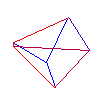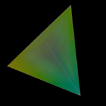### Hypercube

• Also known as the Tesseract
• Dual with cross polytope
• 4D equivalent of the cube
• 8 cubic cells, 24 square faces, 32 edges, 16 vertices
• 3 cubes meet at an edge
• It can be intuitively formed by moving the profile in the current dimension in a direction perpendicular to that dimension by an edge length.
The sequence is: Point - Line - Square - Cube - Hypercube
• Vertices, edges, and faces
• OFF version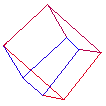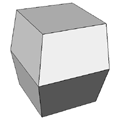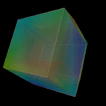### Cross Polytope

• Also known as 16 cell or hexadecachoron
• Dual with hypercube
• 4D equivalent of the octahedron
• 16 tetrahedral cells, 32 triangular faces, 24 edges, 8 vertices
• 4 tetrahedra meet at an edge
• The generation sequence is: Point - Line - Square - Octahedron - Crosspolytope
• Vertices, edges, and faces
• OFF version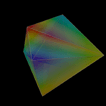### 24 cell

• Also known as the icositetrachoron
• Self-dual
• No equivalent in other dimensions
• 24 octahedral cells, 96 triangular faces, 96 edges, 24 vertices
• 3 octahedra meet at an edge
• Vertices, edges, and faces
• OFF version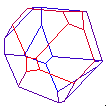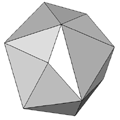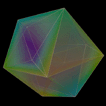### 120 Cell

• Also known as the hecatonicosachoron
• Dual with 600 cell
• 4D equivalent of the dodecahedron
• 120 dodecahedral cells, 720 five sided faces, 1200 edges, 600 vertices
• 3 dodecahedra meeting per edge
• Vertices, edges, and faces
• OFF version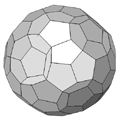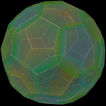### 600 Cell

• Also known as the hexacosichoron
• Dual with 120 cell
• 4D equivalent to the icosahedron
• 600 tetrahedral cells, 1200 triangular faces, 720 edges, 120 vertices
• 5 tetrahedra meeting per edge
• Vertices, edges, and faces
• OFF versionHigher Dimensions

In higher dimensions (5, 6, 7 ....) there are only 3 regular polytopes in any particular dimensions! These 3 regular polytopes are the equivalent of the tetrahedron, cube, and octahedron in 3 dimensions, they are normally called the n-simplex, n-cube, and n-crosspolytope respectively where n stands for the dimension.

n-simplex
• Also known as the hypertetrahedron
• Self-dual
• n + 1 cells each of which is an (n-1) simplex
• n + 1 vertices
• n (n + 1) / 2 edges

n-cube

• Also known as the hypercube
• Dual with n-crosspolytope
• 2 n cells each of which is an (n-1) cube
• 2 n vertices
• n 2 n - 1 edges
• 2 n (n - 1), (n-2) squares

n-crosspolytope

• Also known as the hyperoctahedron
• Dual with n-cube
• 2 n cells each of which is an (n-1) simplex
• 2 n vertices
• 2 n (n - 1) edges
• n 2 n - 1, (n-2) crosspolytopes
Definitions

Dual
The `dual' of a regular polytope is another polytope, also regular, having one vertex in the center of each cell of the polytope we started with. The dual of the dual of a regular polytope is the one we started with (only smaller).

Self-dual
It is possible for a regular polytope to be it's own dual, for example, all the regular polytopes in 2 dimensions. In 3 dimensions the tetrahedron is self-dual. Obviously for a polytope to be self-dual it must have the same number of cells as vertices.

Polyhedral formula
Number of vertices - number of edges + number of faces - number of cells = 0

Vertex/Face ASCII format
These files are formatted as follows, hopefully the description below along with an example from above will give all the information needed to translate the geometry into other formats. For more information see VEF format

• The first line contains the number of vertices
• The next lines (one for each vertex) contain 4 numbers, each consists of the 4 floating point numbers being the w,x,y,z coordinate of that vertex. The line number (counting from 0) is the vertex ID.
• The next line contains the number of edges
• The subsequent lines describe each edge, each consists of two vertex IDs making up that edge.
• The next line contain the number of faces
• The subsequent lines describe each face. The first number of each line is the number of vertices in the face. The rest of the line contains a list of vertex IDs making up that face.
References Regular and Semi-Regular Polytopes. Coxeter, H.S.M. Math. Z. 46, 380-407, 1940.

Experiments in 4 Dimensions. Heiserman, David L. TAB Books Inc

Introduction to Geometry. Coxeter, H.S.M. John Wiley & Sons Inc

4 Dimensional Space Eckhart, L.
Indiana University Press

# 4D objects in VEF format

Sourced mostly from Satoshi Yamaguchi and Gordon Kindlmann
Compiled by Paul Bourke
 120cell.ascii 120cell.off 120cell_1trunc.ascii.gz 120cell_1trunc.off.gz 120cell_snub.ascii.gz 120cell_snub.off.gz 16cell.ascii 16cell.off 16cell_1trunc.ascii 16cell_1trunc.off 24cell.ascii 24cell.off 24cell_1trunc.ascii 24cell_1trunc.off 24cell_snub.ascii.gz 24cell_snub.off.gz 5cell.ascii 5cell.off 5cell_1trunc.ascii 5cell_1trunc.off 5cell_snub.ascii 5cell_snub.off 600cell.ascii 600cell.off 600cell_1trunc.ascii.gz 600cell_1trunc.off.gz 600cell_pluscells.ascii 8cell.ascii 8cell.off 8cell_1trunc.ascii 8cell_1trunc.off 8cell_snub.ascii 8cell_snub.off